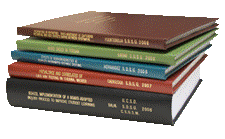Archival Master’s Theses from the University of Nebraska-Lincoln

Thesis

1951

Citation

Thesis (M.A.)—University of Nebraska—Lincoln, 1951. Department of Physics.

Copyright 1951, the author. Used by permission.

Abstract

The problem motivating this study was stated as follows: Given a rocket of specified construction, to determine the performance to be expected of it on the basis of relativistic dynamics. The term “construction” was taken to include the factors exhaust velocity, initial mass, total mass-ratio, and exhaust-rate function as measured on the rocket; “performance” meant the final velocity attained by the rocket and the integrated equation of motion. The scope of the study was limited by the assumptions that the motion was executed in empty space, and that no gravitational fields were present. Only rectilinear motion was considered.

The present study goes beyond previous works in this field primarily in considering the effect of the Einsteinian time-dilation upon the problem of finding an integrated equation of motion.